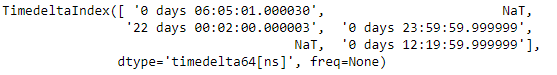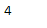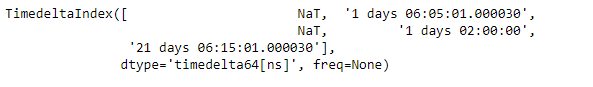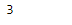# Python | Pandas TimedeltaIndex.nunique

Python is a great language for doing data analysis, primarily because of the fantastic ecosystem of data-centric python packages. Pandas is one of those packages and makes importing and analyzing data much easier.

Pandas` TimedeltaIndex.nunique()` function return number of unique elements in the object. It excludes NA values by default.

Syntax : TimedeltaIndex.nunique()

Parameters :
dropna : Don’t include NaN in the count

Return : nunique : int

Example #1: Use `TimedeltaIndex.nunique()` function to find the number of unique values in the given TimedeltaIndex object.

 `# importing pandas as pd ` `import` `pandas as pd ` ` `  `# Create the TimedeltaIndex object ` `tidx ``=` `pd.TimedeltaIndex(data ``=``[``'06:05:01.000030'``, ``None``, ``'22 day 2 min 3us 10ns'``,  ` `                                   ``'+23:59:59.999999'``, ``None``, ``'+12:19:59.999999'``]) ` ` `  `# Print the TimedeltaIndex object ` `print``(tidx) `

Output :Now we will use the `TimedeltaIndex.nunique()` function to find the number of unique values in the tidx object.

 `# find the number of unique values ` `# in the tidx object. ` `tidx.nunique() `

Output :As we can see in the output, the `TimedeltaIndex.nunique()` function has returned the total number of unique values in the tidx object.

Example #2: Use `TimedeltaIndex.nunique()` function to find the number of unique values in the given TimedeltaIndex object.

 `# importing pandas as pd ` `import` `pandas as pd ` ` `  `# Create the TimedeltaIndex object ` `tidx ``=` `pd.TimedeltaIndex(data ``=``[``None``, ``'1 days 06:05:01.000030'``, ``None``, ` `                       ``'1 days 02:00:00'``, ``'21 days 06:15:01.000030'``]) ` ` `  `# Print the TimedeltaIndex object ` `print``(tidx) `

Output :Now we will use the `TimedeltaIndex.nunique()` function to find the number of unique values in the tidx object.

 `# find the number of unique values  ` `# in the tidx object. ` `tidx.nunique() `

Output :As we can see in the output, the `TimedeltaIndex.nunique()` function has returned the total number of unique values in the tidx object.

My Personal Notes arrow_drop_upCheck out this Author's contributed articles.

If you like GeeksforGeeks and would like to contribute, you can also write an article using contribute.geeksforgeeks.org or mail your article to contribute@geeksforgeeks.org. See your article appearing on the GeeksforGeeks main page and help other Geeks.

Please Improve this article if you find anything incorrect by clicking on the "Improve Article" button below.

Article Tags :

Be the First to upvote.

Please write to us at contribute@geeksforgeeks.org to report any issue with the above content.